# 深度学习笔记4：深度神经网络的正则化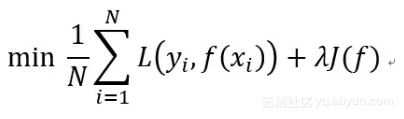L1 和 L2 范数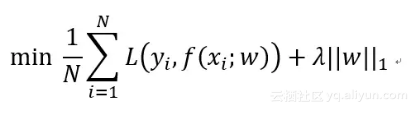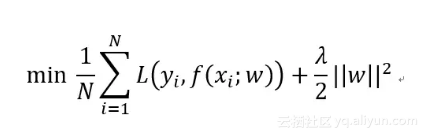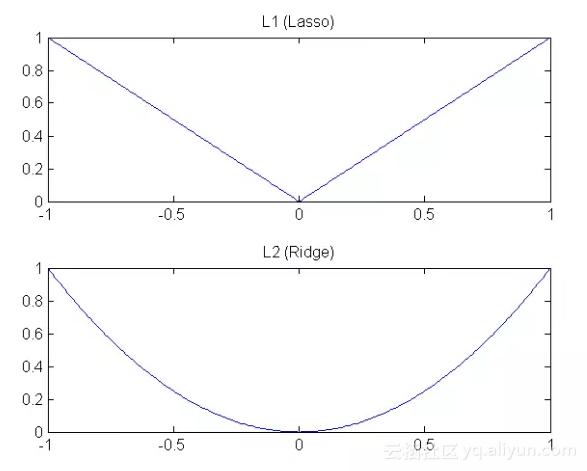L1 和 L2 的下降速度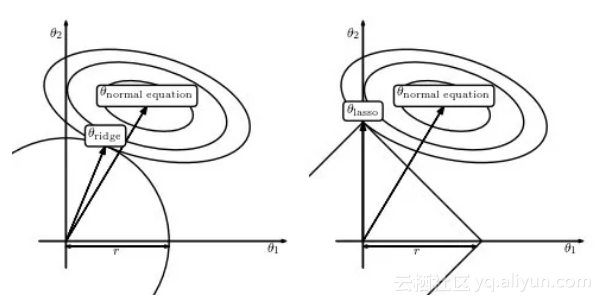L1 和 L2 的模型空间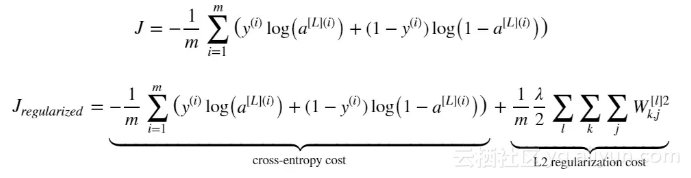def compute_cost_with_regularization(A3, Y, parameters, lambd): """
Implement the cost function with L2 regularization. See formula (2) above.
Arguments:
A3 -- post-activation, output of forward propagation, of shape (output size, number of examples)
Y -- "true" labels vector, of shape (output size, number of examples)
cost - value of the regularized loss function (formula (2))
parameters -- python dictionary containing parameters of the model Returns:
"""
m = Y.shape
W1 = parameters["W1"]
W2 = parameters["W2"]
W3 = parameters["W3"]
cross_entropy_cost = compute_cost(A3, Y) # This gives you the cross-entropy part of the cost
L2_regularization_cost = 1/m * lambd/2 * (np.sum(np.square(W1))+np.sum(np.square(W2))+np.sum(np.square(W3)))

cost = cross_entropy_cost + L2_regularization_cost
return cost

def backward_propagation_with_regularization(X, Y, cache, lambd): """
Implements the backward propagation of our baseline model to which we added an L2 regularization.
Arguments: X -- input dataset, of shape (input size, number of examples)
cache -- cache output from forward_propagation()
Y -- "true" labels vector, of shape (output size, number of examples) lambd -- regularization hyperparameter, scalar Returns:
"""
gradients -- A dictionary with the gradients with respect to each parameter, activation and pre-activation variables

m = X.shape
(Z1, A1, W1, b1, Z2, A2, W2, b2, Z3, A3, W3, b3) = cache
dZ3 = A3 - Y
dW3 = 1./m * np.dot(dZ3, A2.T) + lambd/m * W3
db3 = 1./m * np.sum(dZ3, axis=1, keepdims = True)
dA2 = np.dot(W3.T, dZ3)
dZ2 = np.multiply(dA2, np.int64(A2 > 0))
dW2 = 1./m * np.dot(dZ2, A1.T) + lambd/m * W2
db2 = 1./m * np.sum(dZ2, axis=1, keepdims = True)
dA1 = np.dot(W2.T, dZ2)
dZ1 = np.multiply(dA1, np.int64(A1 > 0))
dW1 = 1./m * np.dot(dZ1, X.T) + lambd/m * W1
db1 = 1./m * np.sum(dZ1, axis=1, keepdims = True)
gradients = {"dZ3": dZ3, "dW3": dW3, "db3": db3,"dA2": dA2, "dZ2": dZ2, "dW2": dW2, "db2": db2, "dA1": dA1,
"dZ1": dZ1, "dW1": dW1, "db1": db1}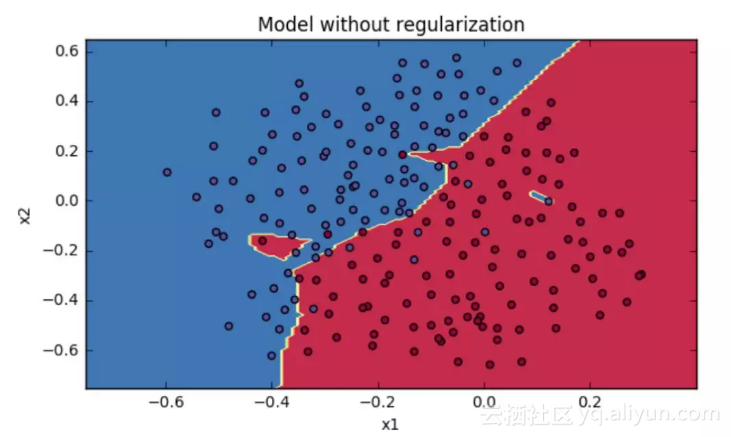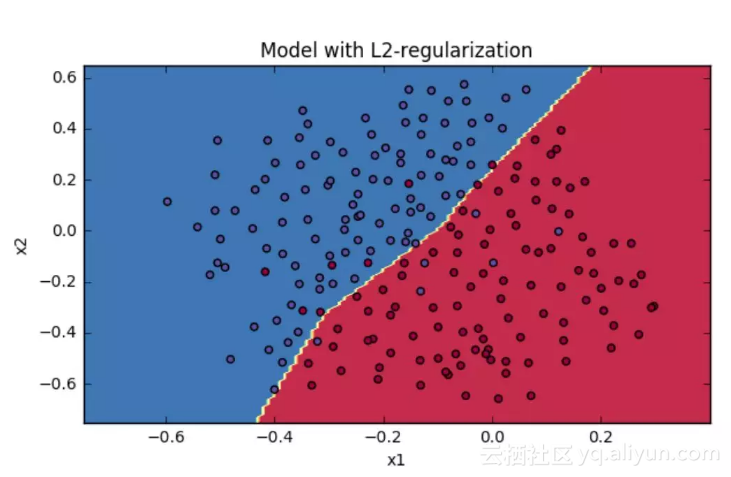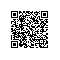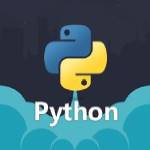Python爱好者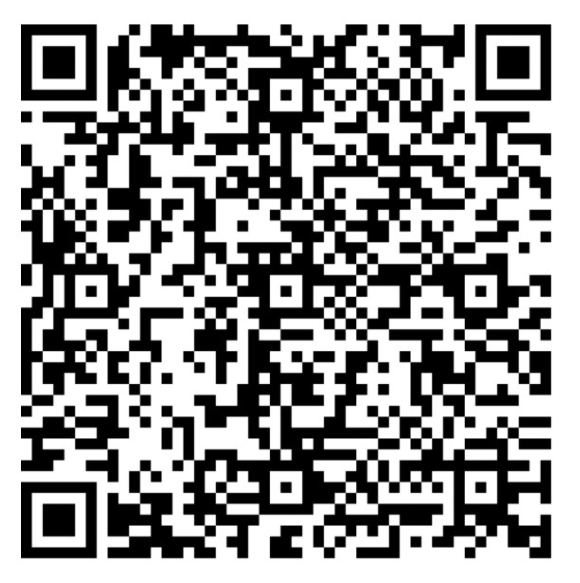使用钉钉扫一扫加入圈子
+ 订阅